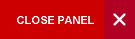## Signals and Systems

Semester 1

This course serves to introduce students to the input-output mode of thinking.  Students will be taught how to translate the everyday notions of cause and effect into input-output models. They will be required to study, understand and use a set of mathematical tools for describing and analyzing inputs and outputs and the relationships between them.   They will study the nature of systems and learn techniques for analyzing and designing them to meet given performance criteria.

The description and use of mathematical tools required to analyze and design systems forms the bulk of the presentation in this course but by appealing to practical examples wherever possible students should never be allowed to lose sight of the real-world application of these tools.

In the presentation of this course and particularly in the tutorials, MATLAB will be used as an omnipresent anchor so that on completion all students should be proficient in its use. The learning experience is enhanced with computer-based exercises and assignments. The assignment will include: (1) take home problem solving questions design to test student understanding of the theory; and (2) a report in the form of an IEEE paper structure on a survey of the state-of-the-art in signal and systems or system engineering. The intent is to expose the student to high level technical publications.

Syllabus:

1. Continuous-Time Signals and Systems

Continuous-Time Elementary Signals: The Unit Step, the Unit Impulse, the Unit Ramp, Sinusoidal Signal

Signal Transformations: Continuity,  Piece-wise continuity; Time shifting, time scaling, time reversal; Convolution; Convolution and Impulse Response

Introduction to Systems: is a system?  Modelling of Physical Systems,  Linear Differential Equations,  I/O State Space; Properties of Systems  (I/O, Linearity, TI,Causality); Testing for System Properties

Frequency Domain Representation of Signals and Systems: The Fourier Series; Trigonometric Form; Complex Exponential Form; Representation of Periodic Signals; Transform

Transform Domain Representation of Systems: Laplace Transfer; System Transfer Function; Block Diagrams; Signal Flow Graphs

Time Domain Analysis of Systems: System Response; Zero Input Response;  Zero State Response; Input-Output Relationships for LTI Systems; and the Impulse Response; The Routh-Hurwitz Criterion; Step Response Analysis; Frequency Response; Space Analysis

2. DISCRETE-TIME SIGNALS AND SYSTEMS

Mathematical Representation of Discrete-Time Signals: Difference Equations; z-Transform; Inverse Transform; Division Z-Transform Inversion; Fraction Expansion; Equations

Frequency Domain Representation of Discrete-Time Signals: Discrete-Time Fourier Transforms; Discrete-Time Fourier Series; Discrete Fourier Transforms; Comparison of Fourier Transforms

Time Domain Representation of Discrete-Time Systems: System Classification; Discrete Time Systems; Discrete Time Convolution;  of Discrete-Time Convolution;  of Discrete-time Systems

Transform Domain Representation of Discrete-Time Systems; Discrete-Time Systems; Stability of Discrete-Time Systems; Time Steady State Response

Filter Design: Analog Filters; Digital Filters (FIR and IIR Filters)

Evaluation:

One 2-hour theory final exam paper – 60%

Mid Semester exam -   20%

Assignments – 20%

• Six take-home problem solving assignment of equal weighting (10%)
• One paper on a survey of the state-of-the-art in the analogue circuit designs (10%). Report will take the form of that required for an IEEE paper publication.
Learning Objectives:

On completion of this course, students should be able to:

• Explain the properties of the different types of continuous time and discrete-time elementary signals and their application to synthesizing complex signals
• Perform test for system characteristics such as linearity, time invariance, causality and memory in both continuous time and discrete time systems
• Explain the convolution theorem in continuous and discrete time form as well as perform graphical convolution of simple continuous time geometric signals and discrete time signals
• Use Fourier Series approximation in all its forms to analyze and synthesize periodic signals as well as use the Fourier Transform to investigate the Frequency Response characteristics of systems
• Apply Laplace Transform techniques to study systems associated with linearity, time invariance, time shifting, scaling, initial value, final value, convolution, differentiation and integration.
• Determine the Transfer Function via Laplace Transform of system’s impulse function and apply it to the construction of  block diagrams of multi-component systems
• Design systems to meet appropriate time domain and frequency domain performance specifications
• Define and determine the z-Transform, inverse z-transform, discrete-time Fourier Series (DTFS) and apply their properties to the design and analysis of electrical filters.
P14A/(PHYS1411 and PHYS1412) and P14B/(PHYS1421 and PHYS1422) and ELET1400 and M08B/MATH0100, M08C/MATH0110 or Equivalent

Text book:   “Signals and Systems: With MATLAB Computing and Simulink Modeling’- by Steven Karris: 4th Edition; Orchard Publications, 2008

Supplemental Reading: Signals, and Systems, Schaum’s Outline Series   Hsu, HweiP, McGraw-Hill, 1997 (3rd Ed)

Internet Resources:

MATLAB TUTORIAL

Signals, and Systems, Schaum’s Outline Series (PDF)

MIT  LECTURE  NOTES PROBLEM SETS AND SOLUTIONS FOR SIGNALS AND SYSTEMS

Course Code:
ELET2460
Credits:
3 Credits
Level:
Level 2
Top of Page